# Input-Output

Input is a group of words, numbers or data that is fed into the system to achieve output or a result, while output means an artifact of the arrangement that has been created by someone or some process.

In this section, the questions are based on a given input of line of words or numbers or numbers or combination of words and numbers that are organised step-by-step and follow a specific rule to arrive at the final step.

Candidates are required to observe the change in order of words or numbers in each step sequentially to specify the rule of arrangement of words and numbers.

Generally, each set of questions on input-output is based on a particular rule. The different sets of questions involve different rules of arrangement of words or numbers.

Most common type of questions on input-output involves arrangement of words as per dictionary or an alphabetical order or arrangement of numbers in ascending or descending order.

Generally, one word or number or both are arranged in every step and procedure is continued until all the words or numbers or both get arranged according to a specific rule.

## Points to be remembered while solving input-output questions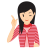MIND IT !

#1: First of all, observe the given input line of words or numbers and the last step of rearrangement, so that candidate may get an idea about the changes in various step of rearrangement.

#2: In order to know what changes have been made in each step, observe two consecutive steps carefully.

#3: Now, correlate the input, the last step and any one of the middle steps. This will enable you to identify the rule of arrangement.

#4: Observe the pattern to know that how many elements are arranged in each step i.e. one or more than one.

#5: The arrangement can be done in any direction, i.e. from left to right to left.

#6: The words can also be arranged according to vowels and consonants.

## Types of Questions

There are mainly four types of questions that are asked from input-output i.e. shifting, arranging, mathematical operations and miscellaneous.

Type #1:
Problem Based on Shifting

In this type of questions, the given words of the input are shifted from one position to another according to a certain set pattern.

Directions (Example Nos. 1-5) Read the following information carefully and answer the questions.
In a SSB interview for the NDA, a group testing officer allocates the following instructions to the candidates divided into groups, who have to perform the tasks according to the input.

Group (I)    Monkey crawling long jump and rope climbing.

Group (II)    Monkey crawling rope jump and long climbing.

Group (III)   Rope crawling monkey climbing long and jump.

Group (IV)   Climbing monkey crawling rope jump and long.

Group (V)    Climbing monkey and rope jump crawling long.

Example: 1

If the task of II group is 'Nothing is without be achieved to determination', then which will be the VI group?

(a) Without is nothing determination to achieved to

(b) Achieved nothing determination to is be without

(c) Nothing is achieved without be to determination

(d) Data is inadequate

(e) None of the above

Example: 2

If 'Terrorism bush job incurbing remarkable did a' is V group task, then which is 'Bush did incurbing remarkable job a terrorism' group?

(a) V

(b) IV

(c) II

(d) VI

(e) None of the above

Example: 3

Asad is first selected for I group "You are my inspiration, guide and instructor", after sometimes he was shifted to group IV. What is his new group task?

(a) Instructor you are and inspiration guide my

(b) You are my guide instructor and inspiration

(c) Inspiration and guide instructor you are my

(d) Data inadequate

(e) None of the above

Example: 4

If the last VII group is "Not job you for this selected are", then which group is I?

(a) You are selected for this not job

(b) Selected for this job you are not

(c) Not you are selected for this job

(d) You are not selected for this job

(e) None of the above

Example: 5

If the task of VI group is "Pollution is becoming cause of deadly diseases", then what is III group?

(a) Pollution is cause of deadly diseases becoming

(b) Diseases becoming is cause of deadly pollution

(c) Becoming is pollution diseases deadly of cause

(d) Data inadequate

(e) None of the above

Solutions (Example Nos. 1-5) The given input follows the pattern shown below.

Group I to II    Based on arrangement of words to different positions. The third word of previous group becomes sixth and sixth become third, interchanging their places.

Group II to III    First three words get reversed and so the group of last four.

Group III to IV  This time we reverse the group of first 4 words and then the last three.

Group IV to V   In group V same rule follows as in group I to group II.

To solve the problems more easily, we can give numbers to each word

Group (I) Monkey
(1)
Crawling
(2)
long
(3)
jump
(4)
and
(5)
rope
(6)
climbing
(7)
Group (II) Monkey
(1)
Crawling
(2)
rope
(6)
jump
(4)
and
(5)
long
(3)
climbing
(7)
Group (III) Rope
(6)
Crawling
(2)
monkey
(1)
climbing
(7)
long
(3)
and
(5)
jump
(4)
Group (IV) Climbing
(7)
monkey
(1)
crawling
(2)
rope
(6)
jump
(4)
and
(5)
long
(3)
Group (V) Climbing
(7)
monkey
(1)
and
(5)
rope
(6)
jump
(4)
crawling
(2)
long
(3)
Group (VI) And
(5)
monkey
(1)
climbing
(7)
long
(3)
crawling
(2)
jump
(4)
rope
(6)
Group (VII) Long
(3)
climbing
(7)
monkey
(1)
and
(5)
rope
(6)
jump
(4)
crawling
(2)

Solution: 1 (b)

Group II-nothing (1) is (2) without (6) be (4) achieved (5) to (3) determination (7). With same logic group VI will be, achieved (5) nothing (1) determination (7) to (3) is (2) be (4) without (6).

Solution: 2 (c)

Group IV-terrorism (7) bush (1) job (5) incurbing (6) remarkable (4) did (2) a (3). It can also be solved by going back but the above system is more convenient. So Bush (1) did (2) incurbing (6) remarkable (4) job (5) a (3) terrorism (7) will be group II.

Solution: 3 (a)

Group I-you (1) are (2) my (3) inspiration (4) guide (5) and (6) instructor (7). Group IV will be, instructor (7) you (1) are (2) and (6) inspiration (4) guide (5) my (3).

Solution: 4 (d)

Last group VII-not (3) job (7) you (1) for (5) this (6) selected (4) are (2). With same logic I group is, you (1) are (2) not (3) selected (4) for (5) this (6) job (7).

Solution: 5 (e)

Group VI-pollution (5) is (1) becoming (7) cause (3) of (2) deadly (4) diseases (6). Group III will be, diseases (6) of (2) is (1) becoming (7) cause (3) pollution (5) deadly (4).

Type #2:
Problem Based on Arrangement

In this type of questions, the numbers or words are arranged as per a given order. The given order can be alphabetical order in case of words and ascending or descending order in case of numbers.

Directions (Example Nos. 6-10) Study the following information carefully and answer the given questions.

A word and number arrangement machine when given an input line of words and numbers rearranges them following a particular rule in each step. The following is an example of input and rearrangement.

Input rose petal 29 32 86 goal 41 toll
Step I 29 rose petal 32 86 goal 41 toll
Step II 29 toll rose petal 32 86 goal 41
Step III 29 toll 32 rose petal 86 goal 41
Step IV 29 toll 32 rose 41 petal 86 goal

Step IV is the last step of rearrangement.

Example: 6

Step III of an input is "15 yes 24 80 today never go 59", which of the following will definitely be the input?

(a) 24 80 today never go 59 15 yes

(b) 24 80 today yes never go 59 15

(c) 24 15 yes 80 today never go 59

(d) Cannot be determined

(e) None of the above

Solution: (d)

Input cannot be determined as we cannot judge the initial position of words and numbers.

Example: 7

Input man 79 over 63 like 43 joy 15. How many step will be required to complete the rearrangement?

(a) Six

(b) Seven

(c) Eight

(d) Nine

(e) None of the these

Solution: (e)

Input man 79 over 63 like 43 joy 15
Step I 15 man 79 over 63 like 43 joy
Step II 15 over man 79 63 like 43 joy
Step III 15 over 43 man 79 63 like joy
Step IV 15 over 43 man 63 79 like joy
Step V 15 over 43 man 63 like 79 joy

So, five steps are required to complete the rearrangement.

Example: 8

Step III of an input is 18 tower 38 basket 82 76 hall new, which of the following will be step VII?

(a) 18 tower 38 hall 76 new basket 82

(b) 18 tower 38 new 76 hall 82 basket

(c) 18 tower 38 hall 76 new 82 basket

(d) There will be no such step

(e) None of the above

Solution: (b)

Step III 18 tower 38 basket 82 76 hall new
Step IV 18 tower 38 new basket 82 76 hall
Step V 18 tower 38 new 76 basket 82 hall
Step VI 18 tower 38 new 76 hall basket 82
Step VII 18 tower 38 new 76 hall 82 basket

So, the correct step VII is given in option (b).

Example: 9

Input 94 join for 81 style home 32 48, which of the following steps will be the last?

(a) VI

(b) V

(c) VII

(d) IX

(e) None of these

Solution: (a)

Input 94 join for 81 style home 32 48
Step I 32 94 join for 81 style home 48
Step II 32 style 94 join for 81 home 48
Step III 32 style 48 94 join for 81 home
Step IV 32 style 48 join 94 for 81 home
Step V 32 style 48 join 81 94 for home
Step VI 32 style 48 join 81 home 94 for

So, last step is step VI.

Example: 10

Step II of an input is 27 world go 57 48 stem 35 kite. How many more steps will be required to complete the rearrangement?

(a) Five

(b) Four

(c) Three

(d) Six

(e) None of these

Solution: (a)

Step II 27 world go 57 48 stem 35 kite
Step III 27 world 35 go 57 48 stem kite
Step IV 27 world 35 stem go 57 48 kite
Step V 27 world 35 stem 48 go 57 kite
Step VI 27 world 35 stem 48 kite go 57
Step VII 27 world 35 stem 48 kite 57 go

So, five more steps are required to complete the rearrangement.

Directions (Example Nos. 11-15) Study the following information carefully and answer the given questions.

When a word and number arrangement machine is a given an input line of words and numbers, it arranges them following a particular rule. The following is an example of input and rearrangement. (All the numbers are two digit numbers)

Input 41 sprain 10 early 97 noble 26 65 ankle death.
Step I 97 41 10 early noble 26 65 ankle death sprain.
Step II noble 97 41 10 early 26 ankle death sprain 65
Step III 41 noble 97 10 26 ankle death sprain 65 early
Step IV death 41 noble 97 10 ankle, sprain 65 early 26
Step V 10 death 41 noble 97 sprain 65 early 26 ankle

Step V is the step of the above arrangement as the intended arrangement is obtained.

As per the rules followed in the given steps, find out the appropriate steps for the given input.

Input omits 36 59 yards 41 elect train 12 lakes 85

Example: 11

Which element comes exactly between 85 and lakes in Step III of the given input.

(a) 59

(b) yards

(c) train

(d) omits

(e) elect

Solution: (e) We have the following arrangement

Input omits 36 59 yards 41 elect train 12 lakes 85
Step I 85 omits 36 59 41 elect train 12 lakes yards
Step II train 85 omits 36 41 elect 12 lakes yards 59
Step III 41 train 85 36 elect 12 lakes yards 59 omits
Step IV lakes 41 train 85 elect 12 yards 59 omits 36
Step V 12 lakes 41 train 85 yards 59 omits 36 elect

'elect' come exactly between '85' and lakes in step III.

Example: 12

If in the first step, '59' interchanges its position with 'lakes' and 'omits' also interchanges its position with '12' then which element will be to the immediate left of '41'?

(a) 59

(b) omits

(c) 12

(d) elect

(e) lakes

Solution: (e) We have the following arrangement

Input omits 36 59 yards 41 elect train 12 lakes 85
Step I 85 omits 36 59 41 elect train 12 lakes yards
Step II train 85 omits 36 41 elect 12 lakes yards 59
Step III 41 train 85 36 elect 12 lakes yards 59 omits
Step IV lakes 41 train 85 elect 12 yards 59 omits 36
Step V 12 lakes 41 train 85 yards 59 omits 36 elect

(e) After interchanging, we get

85 12 36 lakes 41 elect train omits 59 yards.'lakes' is to the immediate left of '41'.

Example: 13

Which of the following combinations represent the first two and the last two elements in the stepV of the given input?

(a) 41, train and omits, 36

(b) 12, lakes and 36, elect

(c) 12, lakes and omits, 36

(d) lakes, 41 and 36, elect

(e) lakes, 41 and 59, omits

Solution: (b) We have the following arrangement

Input omits 36 59 yards 41 elect train 12 lakes 85
Step I 85 omits 36 59 41 elect train 12 lakes yards
Step II train 85 omits 36 41 elect 12 lakes yards 59
Step III 41 train 85 36 elect 12 lakes yards 59 omits
Step IV lakes 41 train 85 elect 12 yards 59 omits 36
Step V 12 lakes 41 train 85 yards 59 omits 36 elect

(b) '12 lakes' are first two and '36 elect' are last two elements in the step V.

Example: 14

Which element is fourth to the right of the one which is ninth from the right end in step V of the give input?

(a) 36

(b) yards

(c) 85

(d) elect

(e) 12

Solution: (b) We have the following arrangement

Input omits 36 59 yards 41 elect train 12 lakes 85
Step I 85 omits 36 59 41 elect train 12 lakes yards
Step II train 85 omits 36 41 elect 12 lakes yards 59
Step III 41 train 85 36 elect 12 lakes yards 59 omits
Step IV lakes 41 train 85 elect 12 yards 59 omits 36
Step V 12 lakes 41 train 85 yards 59 omits 36 elect

(b) 'yards' is fourth to the right of the ninth element from the right end in step V.

Example: 15

In which step are the elements 'train 85 yards 59' found in the same order?

(a) Third

(b) Fourth

(c) Second

(d) Fifth

(e) The given order of elements is not found in any step.

Solution: (d) We have the following arrangement

Input omits 36 59 yards 41 elect train 12 lakes 85
Step I 85 omits 36 59 41 elect train 12 lakes yards
Step II train 85 omits 36 41 elect 12 lakes yards 59
Step III 41 train 85 36 elect 12 lakes yards 59 omits
Step IV lakes 41 train 85 elect 12 yards 59 omits 36
Step V 12 lakes 41 train 85 yards 59 omits 36 elect

(d) 'train 85 yards 59' are found in the same order in step V.

Type #3:
Problem Based on Mathematical Operations

In this type of questions, the input has some numbers. Different steps are obtained by taking the numbers of the input and different arithmetic operations are performed after that.

Directions (Example Nos. 16-20) Study the following information to answer the given questions.

A number arrangement machine, when given a particular input, rearranges it following a particular rule. The following is the example of the input and the steps of the arrangement.

Input 44      35       18    67    22    28    36
Step I 36      27       10    59    14    20    28
Step II 16      15       8      42     4     16    18
Step III 132   105     54    201   66    84    108
Step IV 50      41      24     73    28     34     42
Step V 8         8        9       4         4      1      9
Step VI 64      64       81    169     16  100   81
Step VII 20      19      12      46      8      20    22

Example: 16  Which will be the 4th step of the following input?

Input 24, 88, 22, 34, 81, 90, 38

(a) 30, 94, 28, 40, 87, 92, 40

(b) 30, 94, 28, 40, 87, 96, 44

(c) 44, 96, 87, 40, 28, 94, 30

(d) Cannot be determined

(e) None of the above

Solution: (b) There steps are as follow

Step I (Each number of the input) - 8
Step II Product of the digits of each number of the input.
Step III (Each number of the input) x 3
Step IV (Each number of the input) + 6
Step V Keep adding the digits of each number of the input till they are converted into single digit
Step VI (Digit sum of each number of input)2
Step VII (Each numbers of step II) + 4

(b) Step IV = (Each number of the input) + 6

Example: 17  The second step of a given input is 45, 27, 35, 28, 42, 15. What will be step VII for the input?

(a) 49, 31, 39, 32, 46, 19

(b) 50, 31, 40, 22, 37, 19

(c) 19, 46, 32, 39, 31, 49

(d) Cannot be determined

(e) None of these

Solution: (a) The step are as follow

Step I (Each number of the input) - 8
Step II Product of the digits of each number of the input.
Step III (Each number of the input) x 3
Step IV (Each number of the input) + 6
Step V Keep adding the digits of each number of the input till they are converted into single digit
Step VI (Digit sum of each number of input)2
Step VII (Each numbers of step II) + 4

(a) Step VII = (Each number of step II) + 4

Example: 18  In how many steps would the following arrangement be yielded by the given input?

Input 91, 45, 67, 51, 32, 17

Arrangement 100, 81, 169, 36, 25, 64

(a) VI

(b) III

(c) V

(d) VII

(e) None of these

Solution: (a) The steps are as follow

Step I (Each number of the input) - 8
Step II Product of the digits of each number of the input.
Step III (Each number of the input) x 3
Step IV (Each number of the input) + 6
Step V Keep adding the digits of each number of the input till they are converted into single digit
Step VI (Digit sum of each number of input)2
Step VII (Each numbers of step II) + 4

(a) As given arrangement
= (Digit sum of each number of input)2

Which is the logic of step VI.

Example: 19  What will be the step V of the following input?

Input 37, 48, 91, 22, 49

(a) 10, 12, 10, 4, 13

(b) 4, 4, 1, 3, 1

(c) 1, 3, 1, 4, 4

(d) Cannot be determined

(e) None of the above

Solution: (c) The steps are as follow

Step I (Each number of the input) - 8
Step II Product of the digits of each number of the input.
Step III (Each number of the input) x 3
Step IV (Each number of the input) + 6
Step V Keep adding the digits of each number of the input till they are converted into single digit
Step VI (Digit sum of each number of input)2
Step VII (Each numbers of step II) + 4

(c) As in step V, digits of each number of given input are added till each number get converted into single digit. Let us see

37 => 3 + 7 = 10 => 1 + 0 = 1

48 => 4 + 8 = 12 => 1 + 2 = 3

91 => 9 + 1 = 10 => 1 + 0 = 1

22 => 2 + 2 = 4

49 => 4 + 9 = 13 => 1 + 3 = 4

Example: 20  Find the step III of the following input.

Input 17, 50, 37, 23, 35

(a) 51, 150, 111, 69, 105

(b) 8, 5, 10, 6, 8

(c) 150, 51, 111, 69, 105

(d) Cannot be determined

(e) None of the above

Solution: (a) The steps are as follow

Step I (Each number of the input) - 8
Step II Product of the digits of each number of the input.
Step III (Each number of the input) x 3
Step IV (Each number of the input) + 6
Step V Keep adding the digits of each number of the input till they are converted into single digit
Step VI (Digit sum of each number of input)2
Step VII (Each numbers of step II) + 4

(a) Step III = (Each number of the input) x 3

Directions (Example Nos. 21-25) Study the following information carefully and answer the questions based on it.

An input -output is given in different steps. Some mathematical operation are done in each step.
No mathematical operation is repeated in next step but it can be repeated with some other mathematical operation (as multiplication can be used with subtraction in step 1 and same can be used with addition in step 2).

As per the rules followed in the steps given above, find out in each of the following questions the appropriate step for the given input.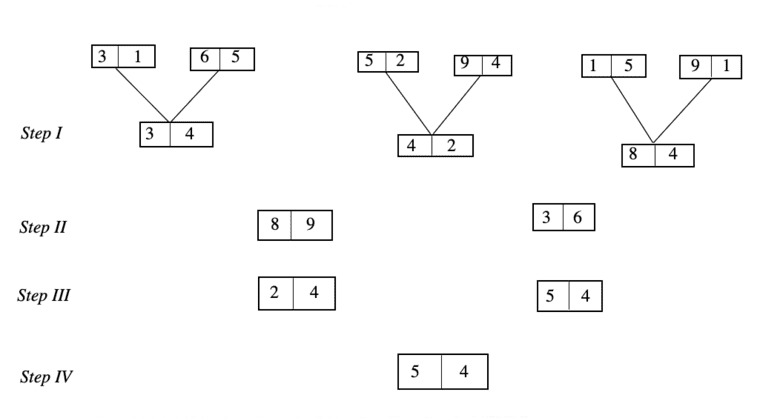As per the rules followed in the steps given above, find out in each of the following questions the appropriate step for the given input.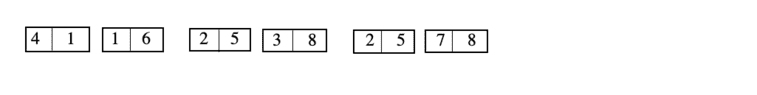Example 21: Find the multiplication of two numbers obtained in step II.

(a) 1409   (b) 1505   (c) 1615   (d) 1705   (e) None of these

Example 22: Find the addition of the three numbers obtained in step I.

(a) 101   (b) 100   (c) 102   (d) 103   (e) None of these

Example 23: Find the difference between the two numbers obtained in step III.

(a) 3   (b) 4   (c) 5   (d) 6   (e) None of these

Example 24: Find the cube of numbers which is obtained in step IV.

(a) 4096   (b) 3375   (c) 6859   (d) 5832   (e) None of these

Example 25: If digit is exchanged within the each block then find the multiplication of two new numbers obtained in step II.

(a) 1500 (b) 1812 (c) 1902 (d) 1802 (e) None of these

Solutions: (Examples 21-25) In the given arrangement, the patterns followed in each step are as follow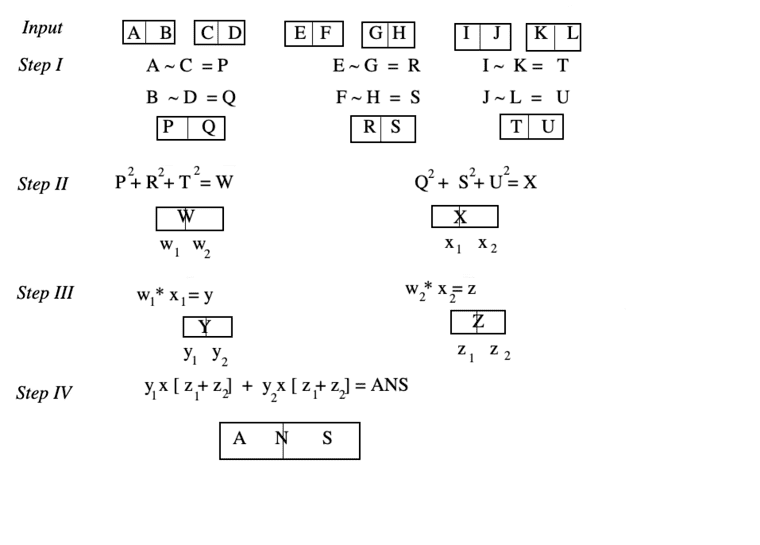Now, the steps of the given input are as follow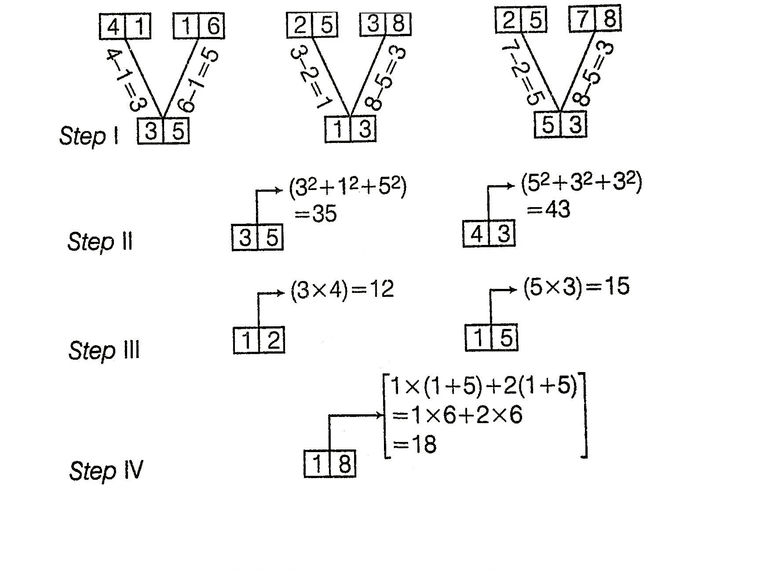Solutions: 21 (b) Required answer = 35 x 43 = 1505

Solutions: 22 (a) Required answer = 35 + 13 + 53 = 101

Solutions: 23 (a) Required answer = 15 - 12 = 3

Solutions: 24 (d) Required answer = (18)3 = 5832

Solutions: 25 (d) Numbers obtained after interchanging the digits 53 and 34Required answer = 1802

Type #4:
Miscellaneous Problems

In this type of questions, the input can be anything and machine performs a set of random operations on this.

Directions (Example Nos. 26-30) Study the following information to answer the given questions.

A number arrangement machine, when given an input line of numbers, rearranges them following a particular rule in each step. The following is an example of input and steps of arrangement.

Input 23  132  38  27   430    287
Step I 2     13    3    2    43      28
Step II 3     32    8    7   30      87
Step III 32  231  83  72  034  782
Step IV 3     2     4      3     5      3
Step V 34  233  49  38  531  388

Example: 26  What will be step V for the following input?

Input 135, 88, 24, 215, 16

(a) 236, 99, 35, 316, 27

(b) 136, 99, 25, 216, 17

(c) 17, 216, 25, 99, 136

(d) Cannot be determined

(e) None of the these

Solution: (a) There steps are as follow

Step I Rightmost digit of each number of input gets disappeared
Step II Leftmost digit of each number of input is disappeared
Step III Leftmost and rightmost digits of each number of input are interchanged
Step IV Leftmost digit of each number of input + 1
Step V Leftmost and rightmost digits of each number of input increase by 1

(a) In step V, leftmost and rightmost digits of each number of input increase by 1.

Example: 27 If step II is 16, 9, 22, 416, 25, 67, then find the input.

(a) 18, 11, 24, 418, 27, 69

(b) 11, 18, 24, 418, 27, 69

(c) 69, 27, 418, 24, 11, 18

(d) Cannot be determined

(e) None of the these

Solution: (a) There steps are as follow

Step I Rightmost digit of each number of input gets disappeared
Step II Leftmost digit of each number of input is disappeared
Step III Leftmost and rightmost digits of each number of input are interchanged
Step IV Leftmost digit of each number of input + 1
Step V Leftmost and rightmost digits of each number of input increase by 1

(d) In step II leftmost digit of each number of input gets disappeared but it is impossible to get leftmost digit of the numbers of input from step II.

Example: 28 What will be the step III for the following input?

Input 777, 29, 435, 115, 61, 37

(a) 777, 29, 436, 116, 62, 37

(b) 777, 92, 534, 16, 511, 73

(c) 777, 92, 534, 511, 16, 73

(d) Cannot be determined

(e) None of the above

Solution: (a) There steps are as follow

Step I Rightmost digit of each number of input gets disappeared
Step II Leftmost digit of each number of input is disappeared
Step III Leftmost and rightmost digits of each number of input are interchanged
Step IV Leftmost digit of each number of input + 1
Step V Leftmost and rightmost digits of each number of input increase by 1

(c) In step III, leftmost and rightmost digits of each number of input are interchanged.

Example: 29 If step I of an input is 4, 16, 121, 8, 17, then find the input.

(a) 45, 163, 1217, 87, 178

(b) 46, 163, 1213, 85, 172

(c) 41, 161, 1216, 82, 176

(d) Cannot be determined

(e) None of the above

Solution: (a) There steps are as follow

Step I Rightmost digit of each number of input gets disappeared
Step II Leftmost digit of each number of input is disappeared
Step III Leftmost and rightmost digits of each number of input are interchanged
Step IV Leftmost digit of each number of input + 1
Step V Leftmost and rightmost digits of each number of input increase by 1

(d) In step I, rightmost digit of each number of input gets disappeared but practically it is impossible to find out rightmost digit of input from step I.

Example: 30  What will be step V for the following input?

Input 220, 197, 15, 37, 89, 75

(a) 3, 2, 2, 4, 9, 8

(b) 1, 8, 6, 8, 10, 6

(c) 6, 10, 8, 6, 8, 1

(d) 8, 9, 4, 2, 2, 3

(e) None of the above

Solution: (a) There steps are as follow

Step I Rightmost digit of each number of input gets disappeared
Step II Leftmost digit of each number of input is disappeared
Step III Leftmost and rightmost digits of each number of input are interchanged
Step IV Leftmost digit of each number of input + 1
Step V Leftmost and rightmost digits of each number of input increase by 1

(a)Step IV = Leftmost digit of each number of input +1

LearnFrenzy provides you lots of fully solved "Input-Output" Questions and Answers with explanation.